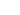# Variables

Variables are a pro feature in ConvertCalculator. They are very similar to formulas. The difference between formulas and variables is that variables can be re-used in other formulas.

If your formulas are simple and straightforward you can get away with re-using bits and pieces in multiple formulas. But you notice that things can get confusing quite fast! We have created Variables to make sure your head won't explode while building a calculator with complex calculations.

Variables act in the same way as Formulas. There is one important difference! A formula is a form element. So formulas are displayed in your calculation form. Variables are only visible if they are used in a formula.

# Creating variables

You can create a variable by expanding the formula editor and clicking on the "Add variable" button. Once you have created the variable, you can use it in any function. You can even nest variables, so you can use variables in other variables. Be careful though about the order in which the variables are defined. Variables that contain a nested variable that is listed below the original variable, will result in an error.# Example

Let's say you have an ROI calculator that calculates "extra revenue", "extra profits" and "return on investment" for your customers. Your product costs \$ 1000,- and you have the following questions:

• `QA`: How much traffic do you have on your website?
• `QB`: How much margin do you get on your product?

You assume that you can bring in \$ 2,- in revenue for every website visitor.

First, you will need to make a variable called `VA: Extra Revenue`. This will look like this:

``QA * 2``

Now you have the "Extra Revenue" variable, you can create a second variable called `VB: Extra Profits`. The formula looks like this:

``VA * QB``

After creating the variables, the formulas look like this:

• Extra Revenue: `VA`
• Extra Profits: `VB`
• Return on Investment: `VB - 1000`